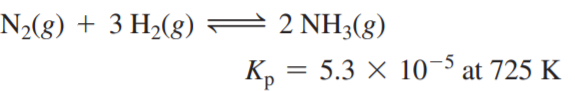×
Get Full Access to Chemistry: A Molecular Approach - 3 Edition - Chapter 14 - Problem 83e
Get Full Access to Chemistry: A Molecular Approach - 3 Edition - Chapter 14 - Problem 83e

×

Ammonia can be synthesized according to the reaction: AISBN: 9780321809247 1

Solution for problem 83E Chapter 14

Chemistry: A Molecular Approach | 3rd Edition

• Textbook Solutions
• 2901 Step-by-step solutions solved by professors and subject experts
• Get 24/7 help from StudySoup virtual teaching assistantsChemistry: A Molecular Approach | 3rd Edition

4 5 1 409 Reviews
22
4
Problem 83E

Ammonia can be synthesized according to the reaction:A 200.0 L reaction container initially contains 1.27 kg of N2 and 0.310 kg of H2 at 725 K. Assuming ideal gas behavior, calculate the mass of NH3 (in g) present in the reaction mixture at equilibrium. What is the percent yield of the reaction under these conditions?

Step-by-Step Solution:

Solution 83EStep 1The given chemical reaction.Equilibrium expression for the given reaction is as follows in terms to pressure.

Step 2 of 9

Step 3 of 9

ISBN: 9780321809247

Chemistry: A Molecular Approach was written by and is associated to the ISBN: 9780321809247. The answer to “?Ammonia can be synthesized according to the reaction: A 200.0 L reaction container initially contains 1.27 kg of N2 and 0.310 kg of H2 at 725 K. Assuming ideal gas behavior, calculate the mass of NH3 (in g) present in the reaction mixture at equilibrium. What is the percent yield of the reaction under these conditions?” is broken down into a number of easy to follow steps, and 56 words. The full step-by-step solution to problem: 83E from chapter: 14 was answered by , our top Chemistry solution expert on 02/22/17, 04:35PM. This full solution covers the following key subjects: reaction, ideal, assuming, behavior, calculate. This expansive textbook survival guide covers 82 chapters, and 9454 solutions. This textbook survival guide was created for the textbook: Chemistry: A Molecular Approach, edition: 3. Since the solution to 83E from 14 chapter was answered, more than 624 students have viewed the full step-by-step answer.

Unlock Textbook Solution